# Inverse function theorem

(diff) ← Older revision | Latest revision (diff) | Newer revision → (diff)
Jump to: navigation, search

## Statement

### Simple version at a specific point

Suppose$f$ is a function of one variable that is a one-one function and$a$ is in the domain of$f$. Suppose$f$ is [differentiable function|differentiable]] at$a$ and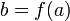$b = f(a)$. Suppose further that the derivative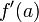$f'(a)$ is nonzero, i.e.,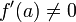$f'(a) \ne 0$. Then:

The inverse function$f^{-1}$ is differentiable at$b$, and further: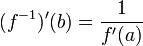$(f^{-1})'(b) = \frac{1}{f'(a)}$

### Simple version at a generic point

Suppose$f$ is a function of one variable that is a one-one function. Then, the formula for the derivative of the inverse function is as follows: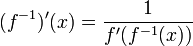$(f^{-1})'(x) = \frac{1}{f'(f^{-1}(x))}$

with the formula applicable at all points in the range of$f$ for which$f'(f^{-1}(x))$ exists and is nonzero.

### One-sided versions

Fill this in later

### Infinity-sensitive versions

Fill this in later